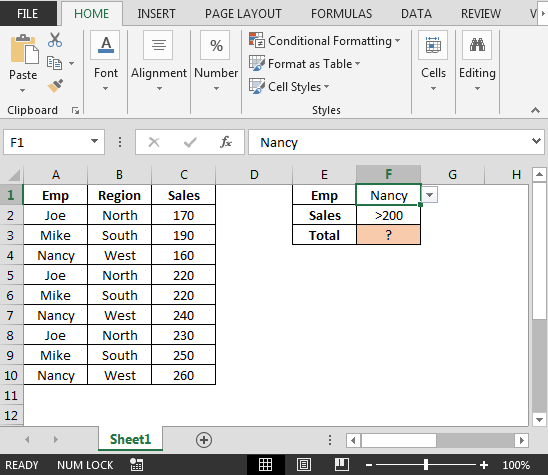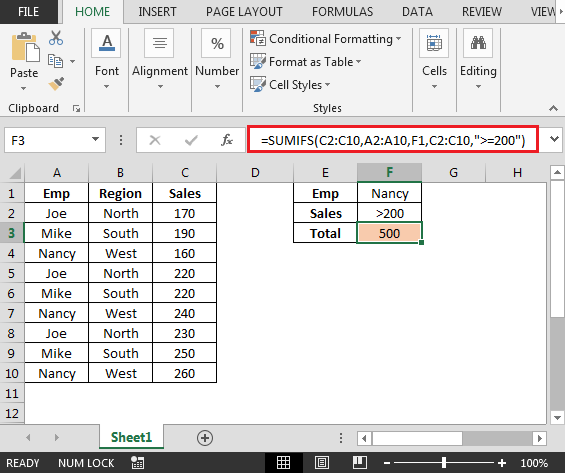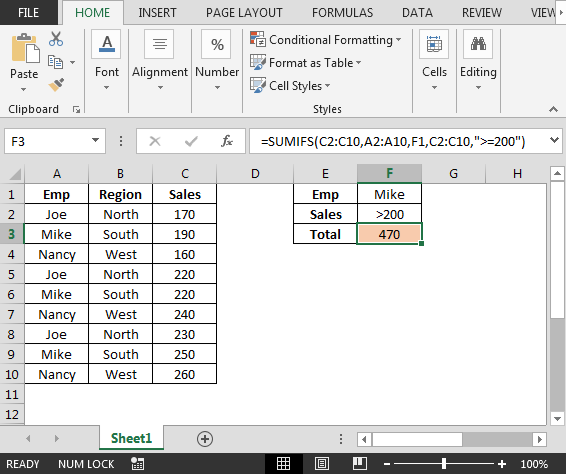# Sumproduct Function with Multiple Columns

In this article, you will learn how to use SUMPRODUCT function with multiple columns. If you have data that contains multiple columns & you want to add the numbers stored in these columns based on the condition (say a code or so).

Let us learn how to use Sumproduct function for adding values in multiple columns.

Following is the snapshot data we have:• In cell I2, the formula is =SUMPRODUCT((A:A="ABC")*B:F)• Sumproduct function is checking the code in column A if found equals to “ABC” then the values will be added for that row.
• Cell A2 does not contain the code hence the values in range B2:F2 will not be added
• In case we change the code in cell A2 to “ABC” then the result be updated automatically.In this way, we can use SUMPRODUCT function to add the sum based on the criteria.